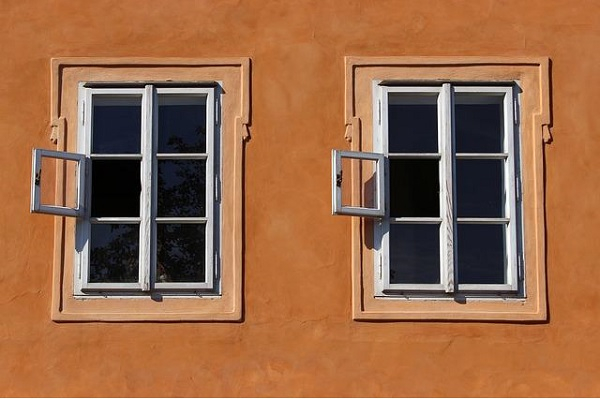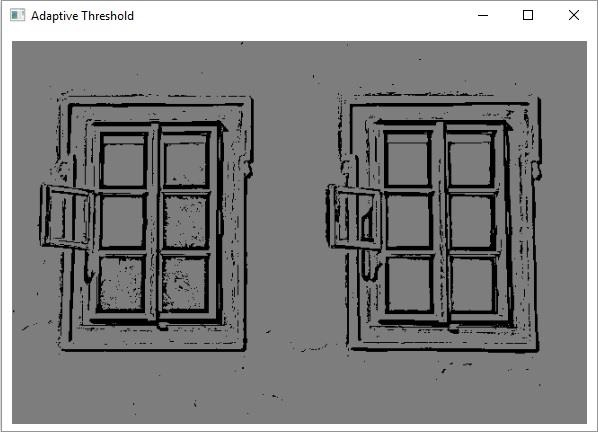# Explain OpenCV Adaptive Threshold using Java Example

Thresholding is a simple technique for the segmentation of an image. it is often used to create binary images. In this, the pixels greater than a given threshold value will be replaced with a standard value.

Adaptive thresholding is the method where the threshold value is calculated for smaller regions and therefore, there will be different threshold values for different regions.

The adaptiveThreshold() method performs the Adaptive Threshold operation on the given image. Following are the parameters of this method −

• Two Mat objects representing the source and destination images.

• An integer variable representing the threshold value.

• Two integer variables representing the adaptive method and threshold type to be used.

• An integer variable type representing the size of the pixel neighborhood used to calculate the threshold value.

• An integer variable of double type representing the constant used in both methods.

## Example

import java.awt.Image;
import java.awt.image.BufferedImage;
import java.io.IOException;
import javafx.application.Application;
import javafx.embed.swing.SwingFXUtils;
import javafx.scene.Group;
import javafx.scene.Scene;
import javafx.scene.image.ImageView;
import javafx.scene.image.WritableImage;
import javafx.stage.Stage;
import org.opencv.core.Core;
import org.opencv.core.Mat;
import org.opencv.highgui.HighGui;
import org.opencv.imgcodecs.Imgcodecs;
import org.opencv.imgproc.Imgproc;
public class AdaptiveThreshold extends Application {
public void start(Stage stage) throws IOException {
String file ="D:\Images\win2.jpg";
//Creating an empty matrices to store the destination image.
Mat dst = new Mat(src.rows(), src.cols(), src.type());
//Applying simple threshold
Imgproc.THRESH_BINARY, 11, 12);
//Converting matrix to JavaFX writable image
Image img = HighGui.toBufferedImage(dst);
WritableImage writableImage= SwingFXUtils.toFXImage((BufferedImage) img, null);
//Setting the image view
ImageView imageView = new ImageView(writableImage);
imageView.setX(10);
imageView.setY(10);
imageView.setFitWidth(575);
imageView.setPreserveRatio(true);
//Setting the Scene object
Group root = new Group(imageView);
Scene scene = new Scene(root, 595, 400);
stage.setScene(scene);
stage.show();
}
public static void main(String args[]) {
launch(args);
}
}

## Input Image## Output

On executing, the above program generates the following windows −Updated on: 13-Apr-2020

562 Views